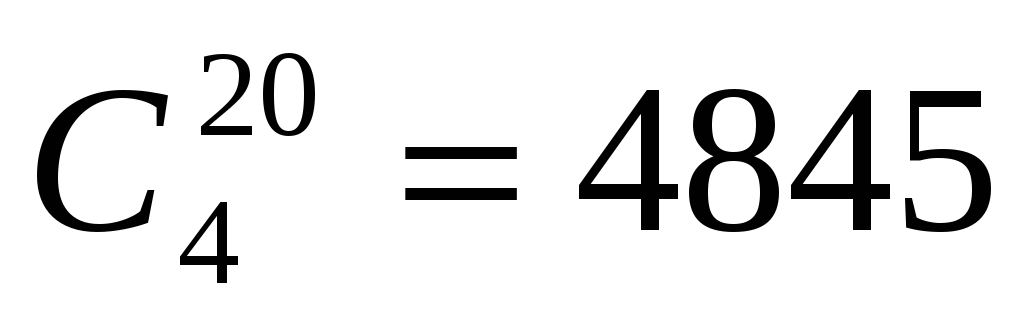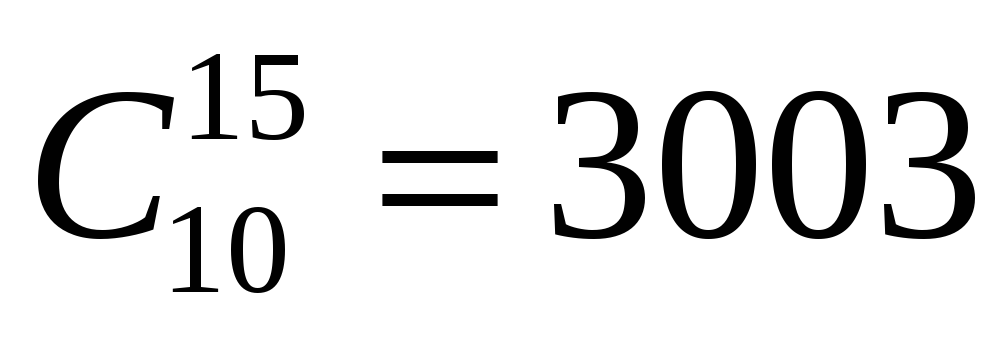# Answer: P(Appearance) = 45% What is the probability that an automobile owner buys a car mainly for its appearance and the automobile owner is a male? answer: P(Appearance and Male) = 23. 75%

Download 145.85 Kb.
 Page 1/2 Date conversion 20.05.2018 Size 145.85 Kb.
1   2
Four hundred randomly sampled automobile owners were asked whether they selected the particular make and model of their present car mainly because of its appearance or because of its performance. The results were as follows:

 Owner Appearance Performance Total Male 95 55 150 Female 85 165 250

What is the probability that an automobile owner buys a car mainly for its appearance?

ANSWER: P(Appearance) = 45%
What is the probability that an automobile owner buys a car mainly for its appearance and the automobile owner is a male?

ANSWER: P(Appearance and Male) = 23.75%
What is the probability that a female automobile owner purchases the car mainly because of its appearance?

ANSWER: P(Appearance | Female) = 34%

A quality-control engineer summarized the frequency of the type of defect with the manufacturing of a certain motor. The following table shows which of the three shifts was responsible for the type of defect:

 Type of Defect Shift Misaligned Component Missing Component Measurement Outside of Specification Limits Other Total 1 23 13 12 14 62 2 15 15 18 12 60 3 5 11 10 2 28

What is the probability that a defective motor will have a measurement outside of its specification limits?

ANSWER: P(Measurement Outside of Specification Limits) = 26.67%
What is the probability that a defective motor was not produced by Shift 1?

ANSWER: P(Shift 1)C = 58.67%
What is the probability that a defective motor produced by Shift 3 does not have a misaligned component?

ANSWER: P(Misaligned Component C | Shift 3) = 82.14%
What is the probability that a defective motor has a misaligned component or was produced by Shift 1?

ANSWER: P(Misaligned Component or Shift 3) = 54.67%
What is the probability that a defective motor was both produced by Shift 2 and the defect was classified as other?

ANSWER: P(Shift 2 and Other) = 8%
What is the probability that a defective motor has a misaligned component and a measurement outside of its specification limits?

ANSWER: P(Misaligned Component and Measurement Outside of Specification Limits) = 0%
Are the events of a motor being produced by Shift 3 and having a defect classified as other independent events?

ANSWER: P(Other | Shift 3) = P(Other) This is a FALSE statement so the events are NOT independent

The employment center at a university wanted to know the proportion of students who worked and also the proportion of those students who lived in the dorm. The following data were collected:

 Work Situation Living Arrangements Full Time Part Time Do Not Work Total In dorm 19 22 20 61 Not in dorm 25 9 5 39 100

What is the probability of selecting a student at random who works either full or part time?

ANSWER: P(FT or PT) = 75%
What is the probability that a student who works lives in the dorm?

ANSWER: P(Dorm | Works) = 54.67%
What is the probability that a student either works full time or does not live in the dorm?

ANSWER: P(FT or Not Dorm) = 58%
Is the event that a student lives in the dorm independent of the event that a student works full time?

ANSWER: P(FT | Dorm) = P(FT) This is a FALSE statement so the events are NOT independent

At a semiconductor plant, 60% of the workers are skilled and 80% of the workers are full-time. Ninety percent of the skilled workers are full-time.

What is the probability that an employee selected at random is a skilled full-time employee?

ANSWER: P(Skilled and FT) = 54%
What is the probability that an employee selected at random is a skilled worker or a full-time workers?

ANSWER: P(Skilled or FT) = 86%
What percentage of the full-time workers are skilled?

ANSWER: P(Skilled | FT) = 67.5%

At a certain university, 30% of the students major in math. Of the students majoring in math, 60% are males. Of all the students at the university, 70% are males.

What is the probability that a student selected at random in the university is a male majoring in math?

ANSWER: P(Male and Math) = 18%
What is the probability that a student selected at random in the university is a male or is majoring in math?

ANSWER: P(Male or Math) = 82%
What proportion of the males are majoring in math?

ANSWER: P(Math | Male) = 25.71%

How many ways can a quality-control inspector select 4 components from a batch of 20 components if the inspector samples without replacement?

ANSWER:How many ways can an advisory board of 10 members be chosen from a list of 15 executives?

ANSWER:How many ways can five employees be assigned to three different shifts?

1   2

The database is protected by copyright ©ininet.org 2016
send message

Main page